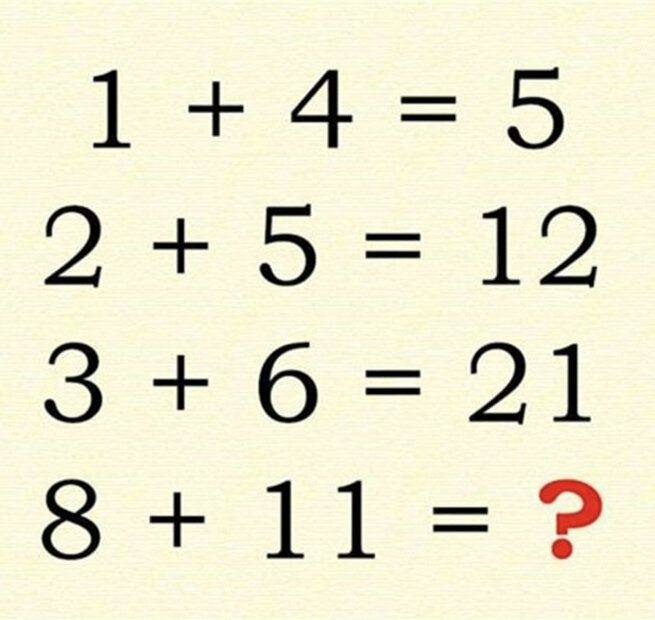# Just for fun: math brain teaser!

• I
whig4life
TL;DR Summary
Just for fun, let me know if I ama+b = a+a*b

1+1*4=5

2+2*5=12

3+3*6=21

8+8*11=96

my friend got 40, which I can also see. I am not sure who is correct. Please let me know and let’s put our heads together and have fun.

Homework Helper
Gold Member
2022 Award
I get ##201##

Mentor
2022 Award
I had 96, too, but there is no unique solution. In fact, there are infinitely many possibilities to construct a building rule.

•sysprog
whig4life
I had 96, too, but there is no unique solution. In fact, there are infinitely many possibilities to construct a building rule.

Hello my friend, and thanks for the reply. How did you arrive at 96, was it similar to my method?

Mentor
2022 Award
Hello my friend, and thanks for the reply. How did you arrive at 96, was it similar to my method?
It was exactly your approach. Appeared to be the easiest way.

Mentor
Summary:: Just for fun, let me know if I am

a+b = a+a*b
That seems the most obvious solution, and one that matches the first three examples.

Mentor
Three other rules that match, using the common b-a=3 in all examples:
##a \oplus b = ab+b-3##
##a \oplus b = a^2+4a##
##a \oplus b = b^2-2b-3##

As the open problem has b-a=3, too, all these rules lead to 96.

Homework Helper
Gold Member
\begin{align} 1 + 4 &= 5_{10} = 5_6 \\ 2 + 5 &= 7_{10} = 12_5 \\ 3 + 6 &= 9_{10} = 21_4 \\ 8 + 11 &= 19_{10} = 201_3 \end{align}